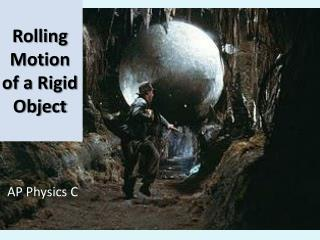DownloadDownload PresentationRolling Motion of a Rigid Object

# Rolling Motion of a Rigid Object

Download Presentation## Rolling Motion of a Rigid Object

- - - - - - - - - - - - - - - - - - - - - - - - - - - E N D - - - - - - - - - - - - - - - - - - - - - - - - - - -
##### Presentation Transcript

1. Rolling Motion of a Rigid Object AP Physics C

2. For pure rolling motion there is “rolling without slipping”, so at point P vp =0. • All points instantaneously rotate about the point of contact between the object and the surface (P).

3. vp’ = 2 vcm

4. Rolling Motion

5. Speed and Acceleration of the CM of a Rolling Object vcm = ωR acm = α R

6. Red Line: Path of a particle on a rolling object (cycloid) Green line: Path of the center of mass of the rolling object

7. http://cnx.org/content/m14374/latest/

8. The Total Kinetic Energy of a Rolling Object is the sum of the rotational and the translational kinetic energy. K = ½ ICMω2 + ½ MvCM2

9. Note • Rolling is possible when there is friction between the surface and the rolling object. • The frictional force provides the torque to rotate the object.

10. Ex: Accelerated Rolling Motion Ki + Ui = Kf + U f Mgh = ½ ICM ω2 + ½ MvCM2 vcm = ωR There is no frictional work. Why not? Does friction cause a displacement at its point of action?

11. Ex: #52 A bowling ball (on a horizontal surface) has a mass M, radius R, and a moment of inertia of (2/5)MR2 . If it starts from rest, how much work must be done on it to set it rolling without slipping at a linear speed v? Express the work in terms of M and v. Hint: use kinetic energy theorem.Ans: (7/10)Mv2

12. Ex: #54 • A uniform solid disk and a uniform hoop are placed side by side at the top of an incline of height h. If they are released from rest and roll without slipping, which object reaches the bottom first? Verify your answer by calculating their speeds when they reach the bottom in terms of h. Use conservation of energy • Ans: The disk, vdisk =(4gh/3)1/2 , vring =(gh)1/2

13. Sample Problem A solid sphere of mass M and radius R rolls from rest down a ramp of height h and angle . Use Conservation of Energy to find the linear acceleration and the speed at the bottom of the ramp.

14. Sample Problem • A solid sphere of mass M and radius R rolls from rest down a ramp of length L and angle q. Use Rotational Dynamics to find the linear acceleration and the speed at the bottom of the ramp.